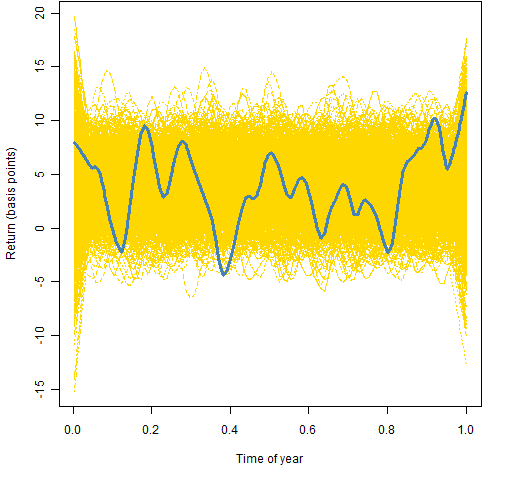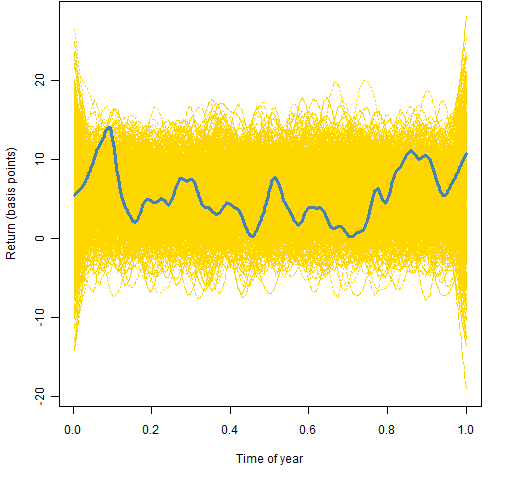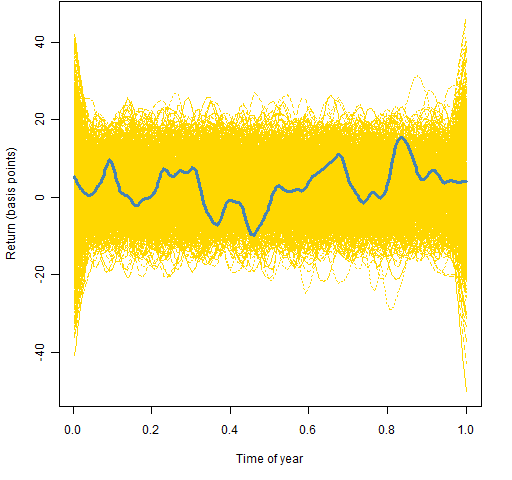Want to share your content on R-bloggers? click here if you have a blog, or here if you don't.

Are there times of the year when returns are better or worse?

Abnormal Returns prompted this question with “SAD and the Halloween indicator” in which it is claimed that the US market tends to outperform from about Halloween until April.

## Data

The data consisted of 15,548 daily returns of the S&P 500 starting in 1950.  Then the point along the year was found for each day.

## Method

The lowess smoother was used to find the typical return for each point in the year.  To see how surprising the deviations are, 1000 instances of permuting the returns (so that there is no trend) were smoothed in the same manner.

## Results

Figure 1 shows the smoothing using the default settings.  As with all the plots, the smooths of the 1000 permuted datasets are shown in thin gold lines, and the smooth of the actual data is shown with a thick blue line.

Figure 1: Lowess smooth with span=2/3 on the full data.Figure 1 is suggestive that the summer has lower returns — consistent with Halloween indicator theory.  However, this is really over-smoothed.  Figure 2 uses a more reasonable level of smoothing.

Figure 2: Lowess smooth with span=0.1 on the full data.With this level of smoothing there appears to be a possibility of a brief bad time in the second quarter, and possibly high values in the fourth quarter.  We can investigate further by breaking the data into two parts — shown in Figures 3 and 4.

Figure 3: Lowess smooth with span=0.1 on the data from 1950 through 1979.Figure 4: Lowess smooth with span=0.1 on the data from 1980 to the present.Figure 4 exhibits the pattern suggested by the Halloween indicator but nowhere near to an extent that should surprise us.  Figure 5 checks to see if the pattern has strengthened lately.

Figure 5: Lowess smooth with span=0.1 on the data from 2000 to the present.The smooth looks to be flatter in this century.

## Summary

The answer to the question in the title seems to be: Probably not.

There have to be periods from the data that are higher than others.  However, to believe that there is a real pattern we should demand that what we observe looks different than random data.  The real data and random data are quite similar in this instance.

## Questions

lowess is handy, but it isn’t really doing the smoothing that we want.  In this case 0 and 1 are the same — we really want to do circular smoothing so that the ends of the year are tied together.  What are the best choices in R for circular smoothing on this sort of data?

Not a qeustion, but: The plots exhibit a known weakness of lowess in that the ends are very variable — going off in straight lines.  lowess should never be used to extrapolate outside the data range.

## Appendix R

#### data update

I already had a vector of S&P 500 returns starting in 1950.  To update that, I did:

require(TTR)
spxnew <- getYahooData('^GSPC', 20100601, 20111017)
spxnewret <- drop(as.matrix(diff(log(spxnew[, 'Close']))))[-1]

The next step was to check that the overlap matched.  Good practice when updating data — especially automatic updates — is to have an overlap and check equality of the overlap.

Then, of course, the two were put together:

spxret <- c(spxret, spxnewret[-1:-21])

#### time of year

Now create the fraction along the year for each day.

spxyears <- substring(names(spxret), 1, 4)
spxyrtab <- table(spxyears)
sp.tlist <- lapply(spxyrtab, function(x) seq(to=x))
spxyrtab <- 252 # current year is only partial
sp.tlist2 <- mapply(`/`, sp.tlist, spxyrtab)
spxseason <- unlist(sp.tlist2)

If you were doing this a lot so that computational time mattered, then there is undoubtedly a clever way of doing this with the data.table package.

#### smoothing and permuting

spx.low <- lowess(spxret ~ spxseason)
lowperm.ymat <- array(NA, c(15548, 1000))
for(i in 1:1000) lowperm.ymat[,i] <- lowess(spxret ~ sample(spxseason))\$y

#### plotting

Figure 1 was created by:

plot(spx.low\$x, spx.low\$y*1e4, xlab="Time of year",  ylab="Return (basis points)", type="n", ylim=1e4 * range(spx.low\$y, lowperm.ymat))

matlines(spx.low\$x, 1e4*lowperm.ymat, col="gold")

lines(spx.low\$x, spx.low\$y*1e4, lwd=3, col="steelblue")

Subscribe to the Portfolio Probe blog by Email